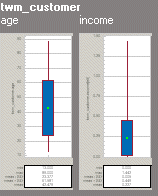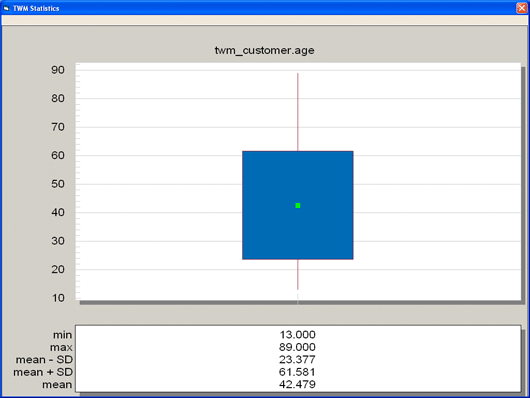# Statistics - Example #1 - Teradata Warehouse Miner

## Teradata Warehouse Miner User Guide - Volume 1Introduction and Profiling

Product
Release Number
5.4.4
Published
July 2017
Language
English (United States)
Last Update
2018-05-03
dita:mapPath
wbc1492033894304.ditamap
dita:ditavalPath
ft:empty
dita:id
B035-2300
Product Category
Software
1. Parameterize a Statistical analysis as follows:
• Columns to Analyze
• twm_customer.income
• twm_customer.age
• Basic Statistics Options
• Number of Values
• Minimum Value
• Maximum Value
• Mean Value
• Standard Deviation
• Skewness
• Kurtosis
• Standard Error
• Coefficient of Variance
• Variance
• Sum
• Uncorrected Sum of Squares
• Corrected Sum of Squares
2. Run the analysis.
3. When it completes, click in the RESULTS tab.

For this example, the Statistical analysis generated the following results. Note that for brevity, the SQL is not shown.

Statistics - Example #1 Data (Part 1)
xtbl xcol xcnt xmin xmax xmean xstd xskew xkurt
TWM_C… income 747 0 144157 22728.2811245 22192.3521561 1.751929 4.3293003
TWM_C… age 747 13 89 42.4792503 19.1020801 .2342409 -.7738404
Statistics - Example #1 Data (Part 2)
xtbl xcol xste xcv xvar xsum xuss xcss
TWM_C… income 811.9757039 97.6420172 492500494.2181 16978026 753779217048 367897869180.964
TWM_C… age .6989086 44.9680253 364.8894624 31732 1620524 272572.4283802
Statistics Example #1 GraphThumbnails for each column in the analyses are shown in a box and whisker plot. The “box” is comprised of a green dot within a blue rectangle. The dot represents the mean value, while the rectangle itself represents plus/minus one standard deviation from the mean. Two “whiskers” are drawn from minus one standard deviation to the minimum value, and from plus one standard deviation to the maximum value.

As the mouse passes over each thumbnail, the cursor changes to indicate a hyperlink, and the individual graphs can be maximized by clicking on the thumbnail:

Statistics Example #1 Graph: Maximize Thumbnail